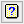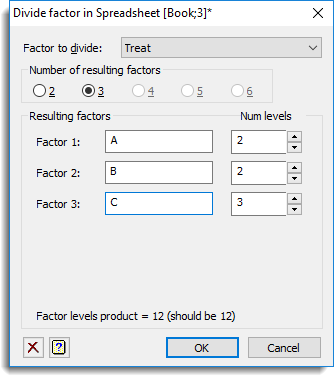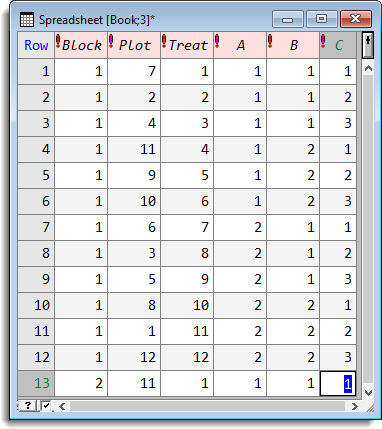1. Home
2. Factor Divide

# Factor Divide

Use this to divide a factor column within a spreadsheet into two or more factors. An example of how dividing a factor may be useful is when generating factors for a design, such as a Latin square, to study several factors and their interactions. For a 12 by 12 Latin square you may have a single factor that you want to divide into two factors A and B with 2 and 4 levels respectively. The factors A and B could be derived by dividing the 12 level treatment factor into two factors with levels (1,1,1,1,2,2,2,2,3,3,3,3) and (1,2,3,4,1,2,3,4,1,2,3,4) respectively.

2. Fill in the fields as required then click OK.The maximum number of factors that can be produced from the divide operation is six. If a factor is to be divided into more than six new factors then use this again on the resulting factors from the previous split. For each factor that is created from the split the name and number of levels must be supplied, also the product of the number of levels of the resulting factors must be the same as the number of levels of factor being divided.

The dialog calculates the divisors for the number of levels in the factor being divided and then these are used to initialize the fields for the number of levels of each factor. If the number of levels does not have enough divisors, then the dialog will not let you specify that number of resulting factors. For example if the number of levels is 12, then at most only 3 factors can be formed as 12 has prime divisors of 2, 2, and 3. If the number of levels of a factor is prime, then a warning will be provided, as this factor may not be divided. The resulting factor columns are added back to the spreadsheet containing the original factor.

Factors can be combined using the factor combine dialog.

## Factor to divide

A dropdown list of the factors in the spreadsheet. Select the factor to be divided into the resulting factors.

## Number of resulting factors

Lets you select the number of factors to divide the factor into (between 2 and 6). If the number of levels of the factor to be divided has at most n prime divisors, then this number must be less or equal to n. On changing this, divisors of n will be entered into the Num Levels input fields.

## Resulting factors

For each of the resulting factors, Factor 1-n, an identifier name and the number of levels (Num Levels) must be supplied in the input fields. The number of levels can be entered in the edit field or selected using the arrows.

## Action IconsClear Clear all fields and list boxes.Help Open the Help topic for this dialog.

## Example

This example shows the Factor Divide dialog being used to split a 12 level factor Treat into two 3 and 4 level factors, A and B. The resulting spreadsheet is shown alongside the dialog.The next example shows the same factor Treat being divided into three 2, 2 and 3 level factors, A, B, and C. The resulting spreadsheet is shown alongside the dialog.